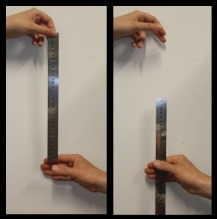#### You may also likeFour vehicles travelled on a road. What can you deduce from the times that they met?### There and Back

Brian swims at twice the speed that a river is flowing, downstream from one moored boat to another and back again, taking 12 minutes altogether. How long would it have taken him in still water?### Escalator

At Holborn underground station there is a very long escalator. Two people are in a hurry and so climb the escalator as it is moving upwards, thus adding their speed to that of the moving steps. ... How many steps are there on the escalator?

# How Do You React?

##### Age 14 to 16Challenge Level

In the problem Reaction Timer, two methods for testing the speed of reactions were introduced.The second experiment suggested getting a friend to drop a ruler and measuring how far along it you could manage to catch. The shorter the distance, the quicker your reactions.

If two people caught the ruler at $15$cm and $30$cm do you think the first person's reactions are twice as fast as the second person's?

To investigate the relationship between the distance the ruler drops and the time taken, we need to do some mathematical modelling. We will assume that:

• the ruler can be modelled as a single particle, rather than something long and thin,
• there is no air resistance on the ruler as it falls.
Objects falling under gravity accelerate at a rate of approximately $9.8$ metres per second per second (usually written as $9.8ms^{-2}$) - that is, each second their velocity increases by $9.8ms^{-1}$.

For an object falling from rest, we can draw a table showing the velocity at regular intervals:

 Time (seconds) 0 1 2 3 4 Velocity (metres per second) 0 9.8 19.6 29.4 39.2

You could represent this graphically - what would the graph look like?

We can work out the distance travelled at each point in time, by multiplying the average velocity by the time taken. Complete the table below:

 Time (seconds) 0 1 2 3 4 Distance travelled (metres)

You could represent this graphically - what would the graph look like?

Using your graph, or by deriving a formula, can you confirm your answer to the question posed at the start of the problem?

The average person's reaction time is about $0.2$ seconds. Where would you expect them to catch a ruler, according to your formula or graph?

Try the experiment for yourself and see if your reaction times are quicker or slower than average.